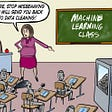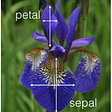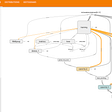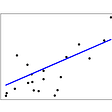# How to write a homemade K Nearest Neighbors model

I am currently in my first half of computer science and for this project, I chose to write a data science algorithm. I got to pick from a list and I chose a knn algorithm. Why? Because it is so easy to build and implement. Working on this project I have a much better understanding of how knn models work.

So to start out, we need to build a rough draft of where we are going with our algorithm.

So the first thing we need to do is create a function that can find the euclidean distance between two vectors. According to Wikipedia, the euclidean distance is defined as, “In mathematics, the Euclidean distance or Euclidean metric is the “ordinary” straight-line distance between two points in Euclidean space. With this distance, Euclidean space becomes a metric space.”

Now that we have our function made, we can start working on building our class.

So in the init function, we are initializing k=3 which means the number of nearest neighbors we are setting to three. After that, we are going to create our fit function, which will be used to fit our data. We can then start on the predict function. We are setting knn_predictions to i. So we are saying, for i in P, run predictions on i. This is where our little helper function comes in. So we are finding the Euclidean distance and then sorting by indices. It is going to return the first item. Then we are going to set our y_train, and then finish by using a counter. What this is doing is finding the single most common item.

So how does this compare to a KNN Classifier from Sklearn?

It is pretty close in its accuracy score, if not the same. I used to iris dataset as it seems to be relatively standard.

Here is the code for the sklearn model (after the data has been processed by train, test, split).

Here is the code for using my knn model using the same processed data.

Here are the predictions for both models. I kept running the code and it keeps outputting the same predictions, so I am happy with the way this turned out.

## More from Jessica Kimbril

28. Data Science Student. Nerd. Lambda School DSPT4

Love podcasts or audiobooks? Learn on the go with our new app.

## Attention: Fourier Transforms. A giant leap in transformer efficiency.## House Prices Prediction using Andrew Ng’s Machine Learning Algorithm## Adaptive Boosting or Ada Boosting algorithm — Hidden Success behind the many Kaggle Competition## Dog Breed Classification using a pre-trained CNN model.## What kind of dog are you?## A Gentle Introduction Into Machine Learning## EDA On IRIS Dataset## Using TensorBoard & Callbacks to Inspect & Monitor Deep Learning Models during Training.## Jessica Kimbril

28. Data Science Student. Nerd. Lambda School DSPT4

## Data Cleaning Journey for Beginners## DEEP LEARNING VS MACHINE LEARNING — DIFFERENCES EXPLAINED## Linear Regression: Important terms to know